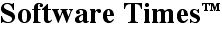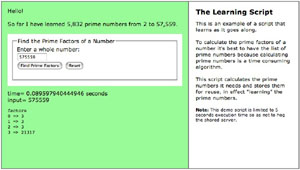Downloads...

March 9, 2013

# The Learning ScriptOne interesting comparison between humans and computers is their ability to learn and store information. Humans are learning machines. We store information internally both genetically and intellectually and we store information externally in what might generally be called "libraries." All the internally stored information disappears on death except for the part that is transmitted genetically to the offspring. The newborn human cannot receive a "download" of information the way a brand new computer can. On the other hand, computers are not generally programed to learn on their own the way humans can and do.

This week I came upon an interesting computer problem, how to calculate the prime factors of numbers. One needs a list of prime numbers to test against. While lists of one hundred and one thousand prime numbers are available, using them is not a general solution because you might need prime numbers larger than what is on these lists. But calculating prime numbers from scratch is time consuming. One solution to this Catch-22 is to have the script or the computer "learn" the prime numbers as they are calculated. In fact this is quite easy to do: every time the script calculates a new, higher prime number it appends it to a prime number file.

## Prime Number Sieve

The script knows "genetically" (is seeded with) the first three prime numbers: 2, 3, and 5 and stores new ones it calculates in a "library" (prime-numbers.php). The following function calculates the prime numbers. It is called as needed. The last thing the function does before exiting is to save the prime number array to a file.
``````
<?phpfunction primeSieve(\$limit, &\$prime, \$url) {    \$max = max(\$prime);       // highest prime number in the array    \$i   = \$max + 2;          // start checking next higher odd number    while(\$limit > \$max) {    // loop from current high prime number to limit        \$test = \$i;           // initialize variables in loop        \$isPrime = TRUE;      // initialize variables in loop        foreach(\$prime AS \$value) {            if (\$test % \$value == 0) {  // if there is an even divisor                \$isPrime = FALSE;       // not a prime number                break;                  // exit foreach loop            }        }        if(\$isPrime) {        // if it is a prime number            \$prime[] = \$i;    // add it to the array            \$max = \$i;        // new highest prime number        }        if (\$i+2 % 5 == 0) {  // if number ends in 5 or 0            \$i += 4;          // increment by 4        } else {              // else            \$i += 2;          // increment by two (don't check even numbers)        }    } // while(\$limit > \$max)        // save prime numbers to file    file_put_contents (\$url, serialize(\$prime));} // function primeSieve()

``````

Give the script a try at The Learning Script (opens in a new window) or download it to see the rest of the code.

Denny Schlesinger

Download The Learning Script (2.9 KB)

Share this article with your followersCopyright © Software Times, 2000 - 2013. All rights reserved.
Last updated March 9, 2013.